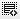## How is net worth calculated?0
How is net worth calculated?1

Net worth is calculated as total assets minus total liabilities of an individual or a company.0

Net worth is the value of all assets, minus the total of all liabilities.0

Total asset - total liabilities0

It is a difference between total asset and total liabilities

Click on thisicon to add code snippet.